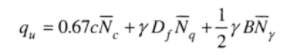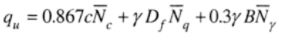# Bearing Capacity Equations for Square, Rectangular and Circular Strip Footings

Terzaghi (1943) developed a rational bearing capacity equation for strip footing, by assuming the bearing capacity failure of the foundation in general shear modeTerzaghi's bearing equation is  given by:

qu = CNc + γ1DfNq + 0.5Bγ2Nγ

• qu= Ultimate Bearing Capacity of the soil
• C= Cohesion
• γ12= Unit weight of the soil above and below the footing level
• Nc,Nq,Nγ= Bearing capacity factors that are a function of friction angle
• Df= Depth of the foundation below the ground level
• B = Width or diameter of the footing
• L= length of the footing

### Bearing Capacity Equation for Square, Rectangular, and Circular Foundations - General Shear Failure

Terzaghi modified the above bearing capacity equation by introducing shape factors for different shapes of the foundation. Then for

#### 1. Square Foundations

qu = 1.3CNc + γDfNq + 0.4BγNγ

#### 2. Circular Foundations

qu = 1.3CNc + γDfNq + 0.3BγNγ

### Bearing Capacity Equation for Square, Rectangular, and Circular Foundations - Local Shear Failure

The above equations were derived from the assumptions of general shear failure. When local shear failure comes into play, the shear parameters in the equations i.e. the c and ф are reduced to a lower limit. Hen e, here instead of c we use Ĉ = 0.67c; and instead of Ф, we use ቔ = 0.67tan
Ф;

The values of NcNq, and Nγ, also change to reduced values that are obtained from Terzaghi's bearing capacity factors for the general shear failure graph, where the bearing capacity factors corresponding to reduced Ф i.e, 0.67tanФ, must be determined.

Then the bearing capacity for:

#### 1. Strip Foundation#### 3. Circular Foundation#### 4. Rectangular Foundation

When the soil is cohesionless, the cohesion factor c = 0; If c =0; the bearing capacity factor Nc = 0; Then the equations for qu can be modified accordingly.

If the soil is cohesive, then the angle of frictional resistance, Ф = 0; then the bearing capacity factors from the graph above, Nγ = 0; Nq= 1; and Nc = 5.7; Based on which the qu equation is determined.